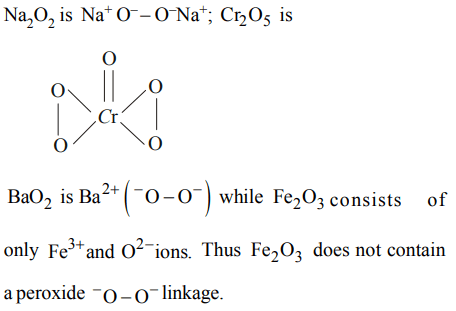## The s-Block Elements Questions and Answers Part-5

1. The stability of the following alkali metal chlorides follows the order
a) LiCl > KCl > NaCl > CsCl
b) CsCl > KCl > NaCl > LiCl
c) NaCl > KCl > LiCl > CsCl
d) KCl > CsCl > NaCl > LiCl

Explanation:2. The order of solubility of lithium halides in non polar solvents follows the order :
a) LiI > LiBr > LiCl > LiF
b) LiF > LiI > LiBr > LiCl
c) LiCl > LiF > LiI > LiBr
d) LiBr > LiCl > LiF > LiI

Explanation: As the size of the anion increases from F to I , the covalent character increase and hence the solubility in non -polar solvent increases LiI > LiBr > LiCl > LiF

3. Which among the following is most soluble in water
a) $CsClO_{4}$
b) $NaClO_{4}$
c) $LiClO_{4}$
d) $KClO_{4}$

Explanation: The high solubility of LiClO4 is mainly due to high heat of hydration of Li+ ion

4. When sodium is treated with sufficient oxygen/air the product obtained is
a) $Na_{2}O$
b) $Na_{2} O_{2}$
c) $NaO_{2}$
d) NaO

Explanation: Na in excess of O2 forms Na2O2

5. Choose the compound which does not possess a peroxide group
a) $Na_{2} O_{2}$
b) $CrO_{5}$
c) $Fe_{2} O_{3}$
d) $BaO_{2}$

Explanation:6. Which of the following alkali metals burns in air to form only monoxide ?
a) Na
b) Li
c) K
d) Cs

Explanation: Li

7. Alkali metals form peroxides and superoxides except
a) Na
b) Rb
c) Li
d) Cs

Explanation: Li+ does not allow its O2- ion to combine with other O atom(s) to form peroxides and superoxides

8. Which is the most basic of the following?
a) $Na_{2}O$
b) BaO
c) $As_{2}O_{3}$
d) $Al_{2}O_{3}$

Explanation: $Na_{2}O$

9. Which of the following is used as a source of oxygen in space capsules, submarines and breathing masks ?
a) $Li_{2}O$
b) $Na_{2}O_{2}$
c) $KO_{2}$
d) $K_{2}O_{2}$a) $K_{2}O$
b) $K_{2}O_{4}$
c) $KO_{3}$
d) $K_{2}O_{3}$